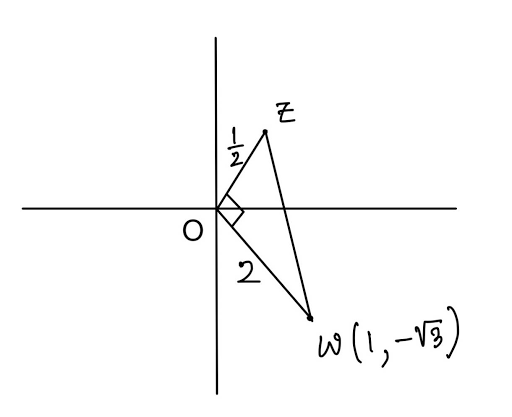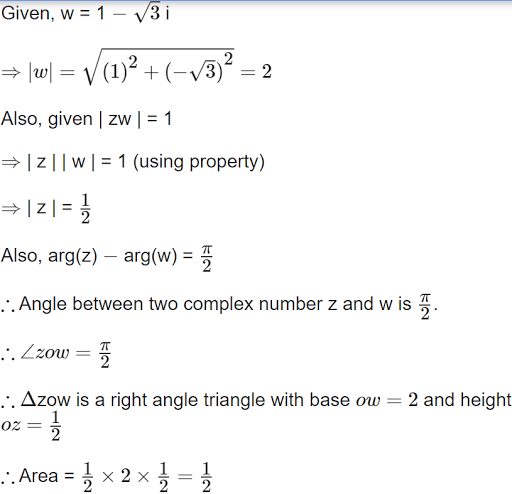25 views

Let a complex number be w = 1 – √3i . Let another complex number z be such that |zw| = 1 and arg(z) – arg(w) = $$\frac{\pi}2$$ . Then the area of the triangle with vertices origin, z and w is equal to :

(1) 4

(2) $$\frac 12$$

(3) $$\frac 14$$

(4) 2

| 25 views

Image::